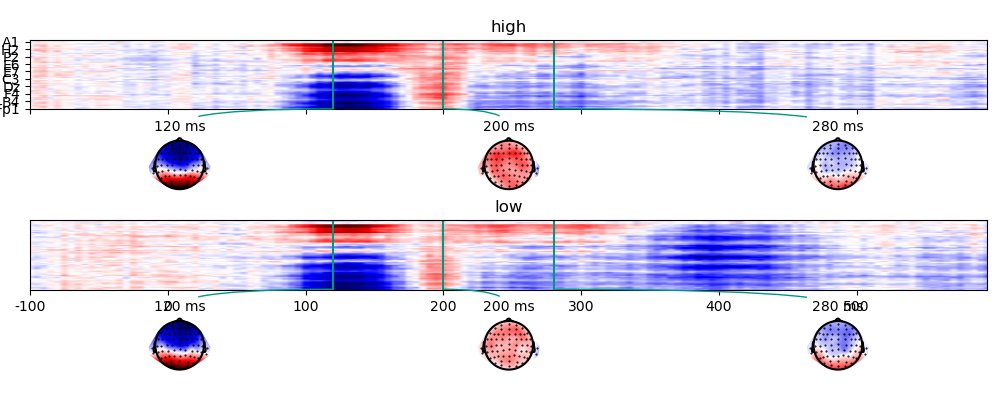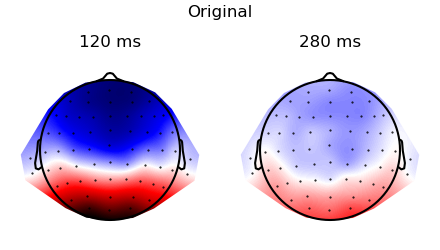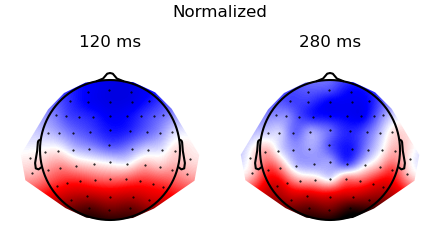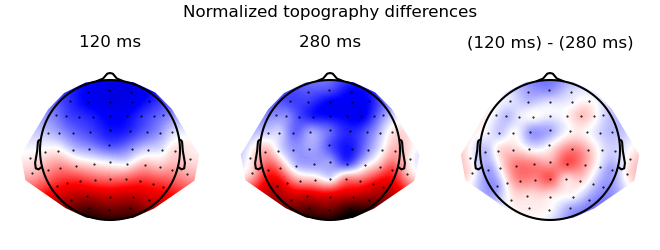# Compare topographies

This example shows how to compare EEG topographies, based on the method described by McCarthy & Wood 1.

```# sphinx_gallery_thumbnail_number = 4
from eelbrain import *
```

## Simulated data

Generate a simulated dataset (as in the T-test example)

```dss = []
for subject in range(10):
# generate data for one subject
ds = datasets.simulate_erp(seed=subject)
# average across trials to get condition means
ds_agg = ds.aggregate('predictability')
# add the subject name as variable
ds_agg[:, 'subject'] = f'S{subject:02}'
dss.append(ds_agg)

ds = combine(dss)
# make subject a random factor (to treat it as random effect for ANOVA)
ds['subject'].random = True
# Re-reference the EEG data (i.e., subtract the mean of the two mastoid channels):
ds['eeg'] -= ds['eeg'].mean(sensor=['M1', 'M2'])
```
```n    cloze     predictability   n_chars   subject
-------------------------------------------------
40   0.88051   high             5         S00
40   0.17241   low              5         S00
40   0.89466   high             4.95      S01
40   0.13778   low              4.975     S01
40   0.90215   high             5.05      S02
40   0.12206   low              4.975     S02
40   0.88503   high             5.2       S03
40   0.14273   low              4.875     S03
40   0.90499   high             5.075     S04
40   0.15732   low              5.025     S04
-------------------------------------------------
NDVars: eeg
```

The simulated data in the two conditions:

```p = plot.TopoArray('eeg', 'predictability', data=ds, ncol=1, axh=2, axw=10, t=[0.120, 0.200, 0.280], head_radius=0.35)
```## Test between conditions

Test whether the 120 ms topography differs between the two cloze conditions. The dataset already includes one row per cell (i.e., per cloze condition and subject). Consequently, we can just index the topography at the desired time point:

```topography = ds['eeg'].sub(time=0.120)
# normalize the data in accordance with McCarth & Wood (1985)
topography = normalize_in_cells(topography, 'sensor', 'predictability', ds)
# "melt" the topography NDVar to turn the sensor dimension into a Factor
ds_topography = table.melt_ndvar(topography, 'sensor', ds=ds)
# Note EEG is a single column, and the last column indicates the sensor
```

ANOVA to test whether the effect of predictability differs between sensors:

```test.ANOVA('eeg', 'predictability * sensor * subject', data=ds_topography)
```

The non-significant interaction suggests that the effect of predictability does not differ between sensors, i.e., the topographies do not differ, which is consistent with being generated by the same underlying neural sources.

## Test two time points

Since we’re not interested in condition here, we first average across conditions, i.e., with the goal of having one row per subject:

```ds_average = ds.aggregate('subject', drop_bad=True)
print(ds_average)
```
```n    cloze     n_chars   subject
--------------------------------
40   0.52646   5         S00
40   0.51622   4.9625    S01
40   0.51211   5.0125    S02
40   0.51388   5.0375    S03
40   0.53115   5.05      S04
40   0.52163   4.9125    S05
40   0.53789   5.0625    S06
40   0.52491   4.8625    S07
40   0.52464   5.2125    S08
40   0.52559   5         S09
--------------------------------
NDVars: eeg
```

In order to compare two time points, we need to construct a new dataset with time point as `Factor`:

```dss = []
for time in [0.120, 0.280]:
ds_time = ds_average['subject',]  # A new dataset with the 'subject' variable only
ds_time['eeg'] = ds_average['eeg'].sub(time=time)
ds_time[:, 'time'] = f'{time*1000:.0f} ms'
dss.append(ds_time)
ds_times = combine(dss)
ds_times.summary()
```

Then, normalize the data in accordance with McCarth & Wood (1985)

```topography = normalize_in_cells('eeg', 'sensor', 'time', data=ds_times)
# "melt" the topography NDVar to turn the sensor dimension into a Factor
ds_topography = table.melt_ndvar(topography, 'sensor', ds=ds_times)
# Note EEG is a single column, and the last column indicates the sensor
```

Plot the topographies before and after normalization:

```p = plot.Topomap('eeg', 'time', data=ds_times, ncol=2, title="Original", head_radius=0.35)
```
••Compre the topographies with the ANOVA – test whether the effect of time differs between sensors:

```test.ANOVA('eeg', 'time * sensor * subject', data=ds_topography)
```

Visualize the difference

```res = testnd.TTestRelated(topography, 'time', match='subject', data=ds_times)```Permutation test:   0%|          | 0/1023 [00:00<?, ? permutations/s]